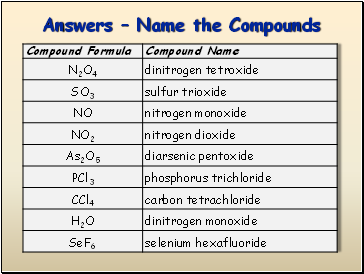# Binary Molecular NomenclaturePage 1

#### WATCH ALL SLIDES

Slide 1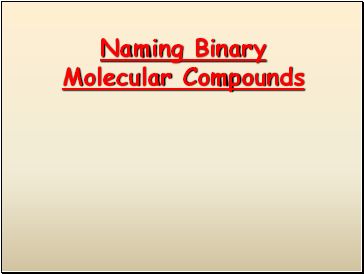Naming Binary Molecular Compounds

Slide 2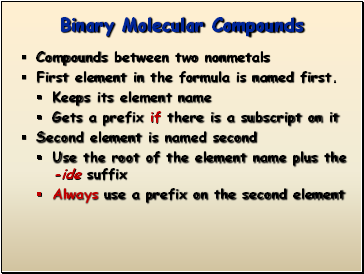## Binary Molecular Compounds

Compounds between two nonmetals

First element in the formula is named first.

Keeps its element name

Gets a prefix if there is a subscript on it

Second element is named second

Use the root of the element name plus the -ide suffix

Always use a prefix on the second element

Slide 3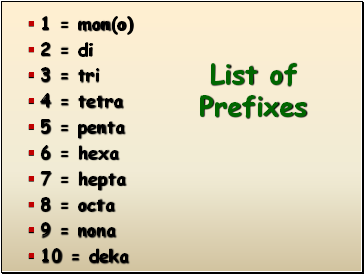List of Prefixes

1 = mon(o)

2 = di

3 = tri

4 = tetra

5 = penta

6 = hexa

7 = hepta

8 = octa

9 = nona

10 = deka

Slide 4## Naming Binary Compounds

P2O5 =

CO2 =

CO =

N2O =

diphosphorus pentoxide

carbon dioxide

carbon monoxide

dinitrogen monoxide

Slide 5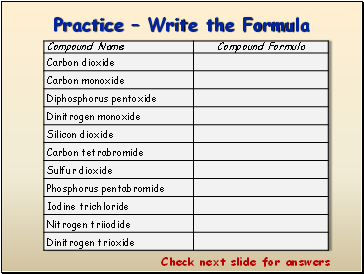Practice – Write the Formula

Slide 6Slide 7Practice – Name the Compounds